# Conversion of Regular Expression to Finite Automata

• Difficulty Level : Medium
• Last Updated : 18 Jan, 2022

As the regular expressions can be constructed from Finite Automata using the State Elimination Method, the reverse method, state decomposition method can be used to construct Finite Automata from the given regular expressions.

Note: This method will construct NFA (with or without ε-transitions, depending on the expression) for the given regular expression, which can be further converted to DFA using NFA to DFA conversion.

### State Decomposition Method

Theorem: Every language defined by a regular expression is also defined by a Finite Automata.

Proof: Let’s assume L = L(R) for a regular expression R. We prove that L = L(M) for some ε-NFA M with:

1) Exactly one accepting state.

2) No incoming edges at the initial state.

3) No outgoing edges at the accepting state.

The proof is done by structural induction on R by following the steps below:

Step 1: Create a starting state, say q1, and a final state, say q2. Label the transition q1 to q2 as the given regular expression, R, as in Fig 1. But, if R is (Q)*, Kleene’s closure of another regular expression Q, then create a single initial state, which will also be the final state, as in Fig 2.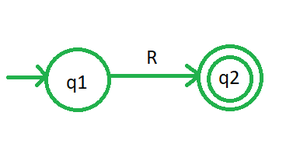Fig 1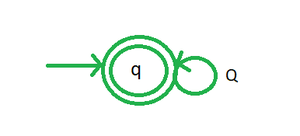Fig 2

Step 2: Repeat the following rules (state decomposition method) by considering the least precedency regular expression operator first until no operator is left in the expression.  Precedence of operators in regular expressions is defined as Union < Concatenation < Kleene’s Closure.

Union operator (+) can be eliminated by introducing parallel edges between the two states as follows.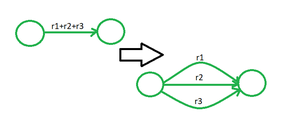Fig 3: Removal of Union Operator

The concatenation operator (‘.’ or no operator at all) can be eliminated by introducing a new state between the states as follows.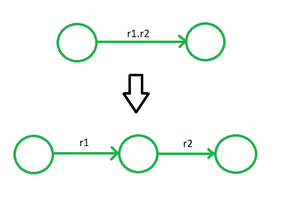Fig 4: Removal of Concatenation Operator

Kleene’s Closure (*) can be eliminated by introducing self-loops on states based on the following conditions:

1. If there is only one outgoing edge at the left-most state, i.e., A in transition A -> B, then introduce self-loop on state A and label edge A to B as an ε-transition, as shown in Fig 5.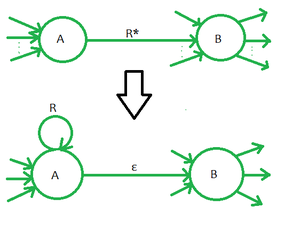Fig 5

2. Else if there is only one incoming edge at the right-most state, i.e., B in transition A -> B, then introduce self-loop on state B and label edge A to B as an ε-transition, as shown in Fig 6.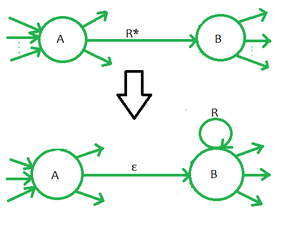Fig 6

3. Else introduce a new state between two states having self-loop labeled as the expression. The new state will have ε-transitions with the previous states as follows, as shown in Fig 7.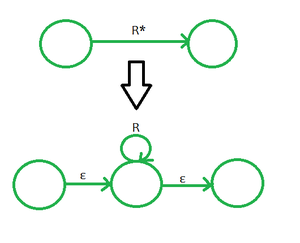Fig 7

### Example:

Construct Finite Automata for the regular expression, R = (ab + ba)*

Solution:

Step 1: As the given expression, R, is of the form (Q)*, so we will create a single initial state that will also be the final state, having self-loop labeled (ab + ba), as shown in Fig 8. (Refer Fig 2 above)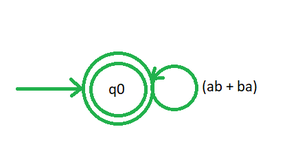Fig 8

Step 2:

A. As the least precedency operator in the expression is a union(+). So we will introduce parallel edges (parallel self-loops here) for ‘ab’ and ‘ba’, as shown in Fig 9.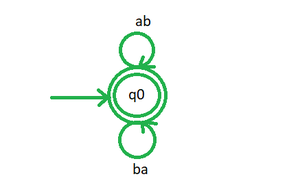Fig 9

B. Now we have two labels with concatenation operators (no operator mentioned between two variables is concatenation), so we remove them one by one by introducing new states, q1 and q2 as shown in Fig 10 and Fig 11. (Refer Fig 4 above)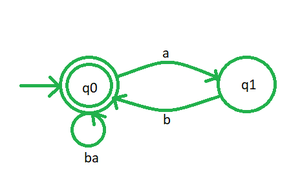Fig 10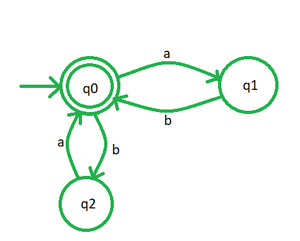Fig 11

Step 3: As no operators are left, we can say that Fig 11 is the required finite automata (NFA).

My Personal Notes arrow_drop_up